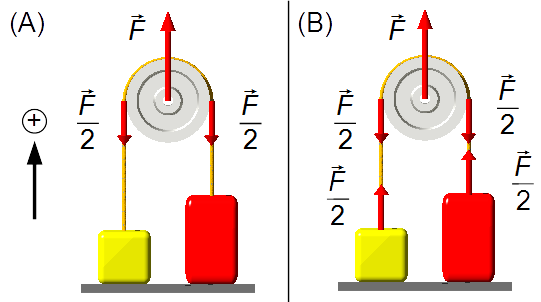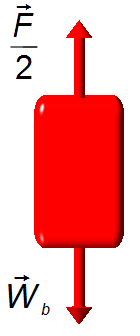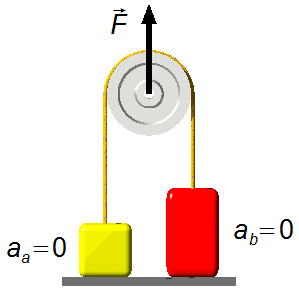Solved Problem on DynamicsPortuguêsEnglish

In an Atwood machine, the two bodies at rest on a horizontal surface, are connected by a cord, of negligible mass, that passes over a frictionless pulley of negligible mass. The masses weight mA = 24 kg and mB = 40 kg and the free-fall acceleration g = 10 m/s2. Find body accelerations when:
a) F = 400 N;
b) F = 720N;
c) F = 1200 N.Problem data
• mass of body A:    mA = 24 kg;
• mass of body B:    mB = 40 kg;
• free fall acceleration:    g = 10 m/s2.
Problem diagram

The frame of reference is oriented positively upward, in the same direction of the force $$\vec F$$.
The force applied to a pulley is evenly distributed between the two sides (figure 1-A), so the magnitude of the force on each side of the pulley will be $$\frac{\vec F}{2}$$.figure 1

Solution

Assuming the rope has a negligible mass it only transmits the force of the pulley to the bodies, thus the component of the force $$\vec F$$ over each body will be $$\frac{\vec F}{2}$$ (figur3 1-B).
Drawing a free-bodies diagram for each block, we have
Body A
• $$\frac{\vec{F}}{2}$$: transmitted force from the pulley;
• $$\vec{P}_{\text{A}}$$: weight of the body A.
The weight of the body A is given byfigure 2
$P_{\text{A}}=m_{\text{A}}g \tag{I}$
Body B
• $$\frac{\vec{F}}{2}$$: transmitted force from the pulley;
• $$\vec{P}_{\text{B}}$$: weight of body B.
The weight of the body B is given byfigure 3
$P_{\text{B}}=m_{\text{B}}g \tag{II}$
Using Newton's Second Law
$\bbox[#99CCFF,10px] {\vec{F}=m\vec{a}}$
we have for body A
$\frac{F}{2}-P_{\text{A}}=m_{\text{A}}a_{\text{A}} \tag{III}$
substituting expression (I) into (III)
$\frac{F}{2}-m_{\text{A}}g=m_{\text{A}}a_{\text{A}} \tag{IV}$
in the same way for body B
$\frac{F}{2}-P_{\text{B}}=m_{\text{B}}a_{\text{B}} \tag{V}$
substituting expression (II) into (V)
$\frac{F}{2}-m_{\text{B}}g=m_{\text{B}}a_{\text{B}} \tag{VI}$

a) If $$F=400\;\text{N}$$, the acceleration of body A will be given by expression (IV)
$\frac{400}{2}-24 \times 10=24 a_{\text{A}}\\ 200-240=24a_{\text{A}}\\ 24a_{\text{A}}=-40\\ a_{\text{A}}=-{\frac{40}{24}}\\ a_{\text{A}}=-1.7\ \text{m}/s^{2}$
For body B we have the expression (VI)
$\frac{400}{2}-40 \times 10=40a_{\text{B}}\\ 200-400=40a_{\text{B}}\\ 40a_{\text{B}}=-200\\ a_{\text{B}}=-{\frac{200}{40}}\\ a_{\text{B}}=-5\ \text{m}/s^{2}$
As the accelerations are negative, the bodies must move against the direction of the frame (downward), but as they are on a surface they remain at rest and their accelerations are zero

$\bbox[#FFCCCC,10px] {a_{\text{A}}=a_{\text{B}}=0}$figure 4

b) If $$F=720\;\text{N}$$, the acceleration of body A will be given by expression (IV)
$\frac{720}{2}-24 \times 10=24a_{\text{A}}\\ 360-240=24a_{\text{A}}\\ 24a_{\text{A}}=120\\ a_{\text{A}}=\frac{120}{24}\\ a_{\text{A}}=\;5\ \text{m}/s^{2}$
For body B we have the expression (VI)
$\frac{720}{2}-40 \times 10=40a_{\text{B}}\\ 360-400=40a_{\text{B}}\\ 40a_{\text{B}}=-40\\ a_{\text{B}}=-{\frac{40}{40}}\\ a_{\text{B}}=-1\ \text{m}/s^{2}$
The body A has the acceleration

$\bbox[#FFCCCC,10px] {a_{\text{A}}=5\ \text{m}/s^{2}}$

As the acceleration of the body B is negative, it must move against the orientation of the frame (downward), but as it is on a surface it remains at rest and its acceleration will be zero

$\bbox[#FFCCCC,10px] {a_{\text{B}}=0}$figure 5

c) If $$F=1200\;\text{N}$$, the acceleration of body A will be given by the expression (IV)
$\frac{1200}{2}-24 \times 10=24a_{\text{A}}\\ 600-240=24a_{\text{A}}\\ 24a_{\text{A}}=360\\ a_{\text{A}}=\frac{360}{24}\\ a_{\text{A}}=\;15\ \text{m}/s^{2}$
For body B we have the expression (VI)
$\frac{1200}{2}-40 \times 10=40a_{\text{B}}\\ 600-400=40a_{\text{B}}\\ 40a_{\text{B}}=200\\ a_{\text{B}}=\frac{200}{40}\\ a_{\text{B}}=5\ \text{m}/s^{2}$
Body A has acceleration

$\bbox[#FFCCCC,10px] {a_{\text{A}}=15\ \text{m}/s^{2}}$

And body B has acceleration

$\bbox[#FFCCCC,10px] {a_{\text{B}}=5\ \text{m}/s^{2}}$figure 6